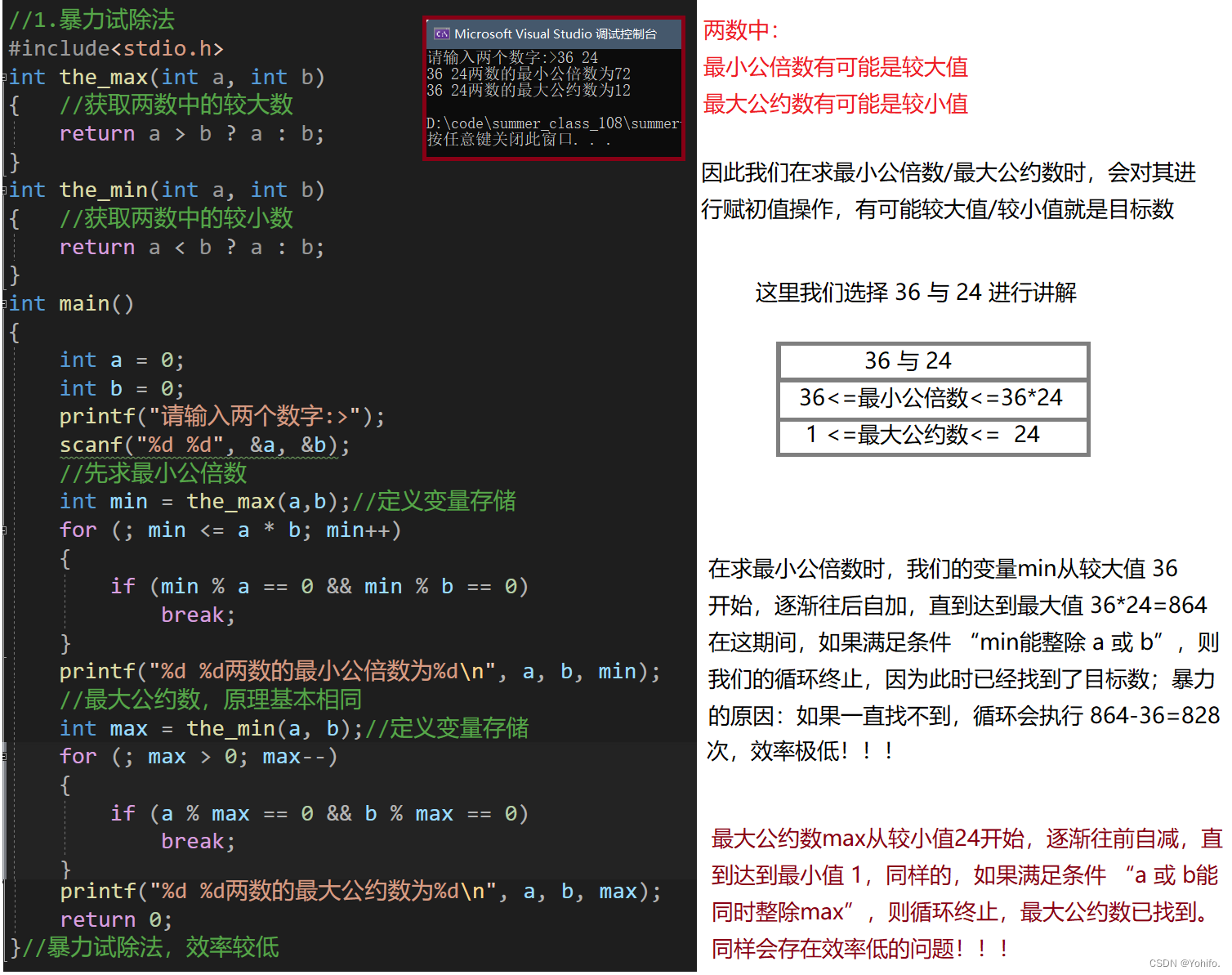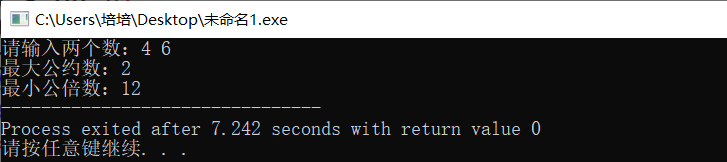# 更相损减法和辗转相除法 求最大公约数和最小公倍数（C语言）

+关注继续查看

m为最大公约数

a=m*i; b=m*j;

《九章算術·方田》作分數約簡時，提到求最大公因數方法：反覆把兩數的較大者減去較小者，直至兩數相等，這數就是最大公因數。這方法除了把除法換作減法外，與輾轉相除法完全相同。例如書中求91和49的最大公因數：

1. 91 > 49, 91 - 49 = 42
2. 49 > 42, 49 - 42 = 7
3. 42 > 7, 42 - 7 = 35
4. 35 > 7, 35 - 7 = 28
5. 28 > 7, 28 - 7 = 21
6. 21 > 7, 21 - 7 = 14
7. 14 > 7, 14 - 7 = 7
8. 7 = 7, 因此91和49的最大公因數是7

1. ra ÷ b 的餘數, 則

gcd(a,b) = gcd(b,r)

1. a 和其倍數之最大公因數為 a

1. a ÷ b，令r為所得餘數（0≤rb

r = 0，演算法結束；b 即為答案。

1. 互換：置 abbr，並返回第一步。

function gcd(a, b) {

if a mod b<>0

return gcd(b, a mod b);

else

return a;

}

或纯使用循环:

function gcd(a, b) {

define r as integer;

while b ≠ 0 {

r := a mod b;

a := b;

b := r;

}

return a

}

其中“a mod b”是指取 a ÷ b 的余数。

C语言：

#include<stdio.h>

int gcd(int a,int b)//最大公约数

{

if (a<b) return gcd(b,a);

else if (b==0) return a;

else return gcd(b,a%b);

}

int lcm(int a,int b)

{

return a*b/gcd(a,b);

}

main()

{

int a,b;

scanf("%d%d",&a,&b);

printf("最大公约数：%d\n",gcd(a,b));

printf("最小公倍数：%d\n",lcm(a,b));

}

#include int main()
{
int m, n; int m_cup, n_cup, res;
printf("Enter two integer:\n");
scanf("%d %d", &m, &n);
if (m > 0 && n>0)
{
m_cup =m;
n_cup =n;
res = m_cup% n_cup;
while (res!= 0)
{
m_cup = n_cup;
n_cup = res;
res = m_cup % n_cup;
}
printf("Greatestcommon divisor: %d\n", n_cup);
printf("Leasecommon multiple : %d\n", m * n / n_cup);
}
else printf("Error!\n");
return 0;
}C语言题解——最小公倍数的三种求法（含最大公约数）

89 0C语言求两个数最大公约数和最小公倍数
C语言求两个数最大公约数和最小公倍数
17 0（第23列）C语言典型题：求两个数的最小公倍数和最大公约数。（两种解法）
（第23列）C语言典型题：求两个数的最小公倍数和最大公约数。（两种解法）
60 059 0C语言基础-编写求两个整数最大公约数的函数，并调用此函数求两个整数的最大公约数和最小公倍数。（头文件、方法主体、主函数，分离）

180 0c语言：输入两个正整数 求最大公约数和最小公倍数
c语言：输入两个正整数 求最大公约数和最小公倍数
2202 0C语言：输入两个数，输出最大公约数，最小公倍数
1267 0c语言求最小公倍数，最大公约数
1547 0C语言求最小公倍数和最大公约数三种算法(经典)

1309 0C语言OJ项目参考(1047) 最大公约数和最小公倍数
1047: 求两个整数的最大公约数和最小公倍数 Description 写两个函数，分别求两个整数的最大公约数和最小公倍数，用主函数调用这两个函数，并输出结果两个整数由键盘输入。 Input 两个数 Output 最大公约数 最小公倍数 Sample Input 6 15 Sample Output 3 30 HINT 主函数已给定如下，提交时不需要包
1411 0

C语言学习教程189641281534044